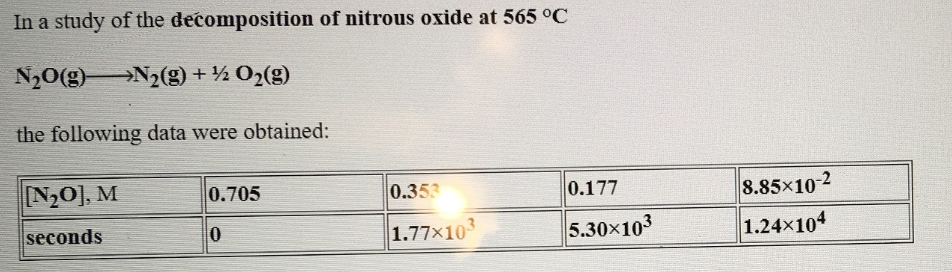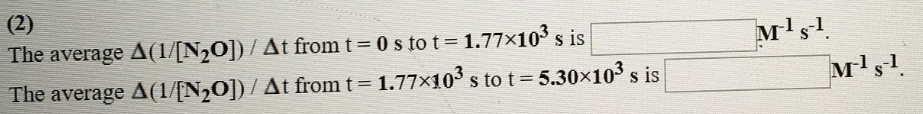# In a study of the decomposition of nitrous oxide at 565 °C N2O(g) → N2(g) + 1/2 O2(g) The following data were obtained: The average Δ ( 1/[N2O]) / Δt from t = 0 s to t = 1.77x10^3 s is ________ M^-1 s^-1. The average Δ ( 1/[N2O]) / Δt from t = 1.77x10^3 s to t = 5.30x10^3 s is _______ M^-1 s^-1. .